Math Concepts

# Introduction to Complex Numbers

718 views

Table of Contents

 1 Introduction 2 Definition 3 What are Complex Numbers? 4 What are Real Numbers and Imaginary Numbers? 5 Algebraic Operations on Complex Numbers 6 Complex Number Formulas 7 Power of iota 8 Complex Number Identities 9 Modulas and Conjugate 10 Summary 12 Frequently Asked Questions (FAQs)

15th September'20

Reading time: 10mins

The English scientist G.H. Hardy remarked that Gauss was the primary scientist to use complex numbers in a precisely assured and scientific approach.

Several mathematicians contributed to the event of complex numbers. The foundations for addition, subtraction, multiplication, and root expression of complex numbers developed by the Italian scientist Rafael Bomb Elli. A lot of conspectus formalism for the complex numbers was developed by the Irish mathematician William Rowan Hamilton, who extended this contemplation to the speculation of quaternions.

Later classical writers on the overall theory embraced Richard Dedekind, Otto Holder, Felix Klein, Henri Poincare, Herman Schwarz, Karl Weierstrass, and lots of others.

## Definition of Complex Numbers

Complex numbers expressed within the variety of a + ib where i is an imaginary number is known as iota and has the worth of (√-1). An example, 2 + 3i may be a complex quantity, wherever a pair of maybe an imaginary number and 3i is a complex quantity. Therefore, the mixture of each real number and imaginary number may be a complex number.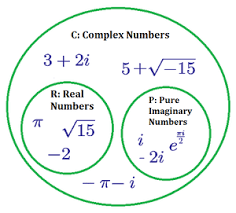The main application of these numbers is to represent periodic motions like water waves, AC, light-weight waves, etc. These depend on trigonometric function or trigonometric function waves, etc. There are sure formulas that are to solve the issues supporting complex numbers. Also, mathematical operations like addition, subtraction, and multiplication are performed on these numbers.

Download the PDF below to understand the definition and basic properties of Complex Numbers.

 📥 Introduction to Complex Numbers Download

## What are Complex Numbers?

The complex number consists of a real number and an imaginary number. The complex quantity is of the shape a + ib. The real numbers are the numbers that we tend to sometimes work on to try and do the mathematical calculations. The imaginary numbers don't seem to be typically used for calculations solely within the case of imaginary numbers allowing us to check the definitions for each of the numbers.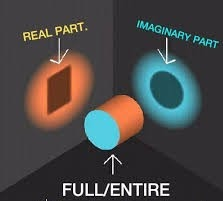## What are Real Numbers?

Any variety that is a gift in an exceeding system of numeration like positive, negative, zero, integer, rational, irrational, fractions, etc. are real numbers. It is pictured as Re (). For example, 12, -45, 0, 1/7, 2.8, √5, etc., are all real numbers.

## What are Imaginary numbers?

The numbers that don't seem to be real are imaginary. When we square an imaginary quantity provides a negative result. It is pictured as im (). Example: √-2, √-7, √-11 are all imaginary numbers.
The complex number introduced to resolve the equation x2+1 = 0. The roots of the equation are of kind x = ±√-1 never real root exists. Thus, with the introduction of complex numbers, we've got imaginary roots. It denotes √-1 with the image i where i denotes iota (Imaginary number).
An equation of the shape z= a + i b, wherever a and b are real numbers, is outlined to be a fancy variety of complex numbers. The actual half denoted by Re z = a. The imaginary part of a complex number as im z = ib.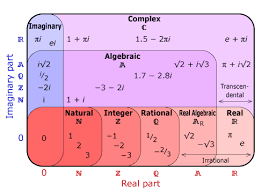See the table below to differentiate between an actual variety and a complex quantity.

Complex Number Real Number Imaginary Number
-1+2i -1 2i
7-9i 7 -9i
-6i 0 (Purely Imaginary) -6i
6 6 0 (Purely Real)

## Algebraic Operations on Complex Numbers

There are four sorts of pure mathematics operations on complex numbers they mentioned below. Let's understand more regarding these pure mathematics operations together with solved examples. The four operations on the complex numbers include:

•  Addition
•  Subtraction
•  Multiplication
•  Division

### Roots of Complex Numbers:

When we solve a quadratic within the variety of ax2 +bx +c = 0, the root of the equations determined in 3 forms;

• Two Distinct Real Roots
•  Similar Root
•  No Real roots (Complex Roots)

## Complex Number Formulas

While doing any activity on the arithmetic operations of complex numbers like addition and subtraction, mix similar terms. It implies that a mix of the real numbers with the actual number and imaginary number with the imaginary number.

### Addition:

(a + ib) + (c + id) = (a + c) + i(b + d)

### Subtraction:

(a + ib) – (c + id) = (a-c) + i(b-d)

### Multiplication:

When 2 complex numbers are increased by one another, the multiplication method ought to be almost like the multiplication of 2 binomials. It implies that the FOIL technique (Distributive multiplication process) is employed.
(a + ib) x (c + id) = (ac – bd) + i(ad + bc)

### Division:

The division of 2 complex quantities is performed by multiplying the dividend and divisor by its conjugate worth of the divisor, so apply the FOIL technique.
(a + ib) / (c + id) = (ac +bd)/ (c2 + d2) +i (bc – ad) / (c2 + d2)

## Power of Iota (i)

Depending upon the ability of “i”, it will take the subsequent values;
i4k+1 = i.i4k+2 = -1 i4k+3 = -i. i4k = 1
Where k will have an integral value (positive or negative).
Similarly, we are able to notice for the negative power of i, that are as follows; i-1 = 1 / i
Multiplying and dividing the above of term with i, we have;
i-1 = 1 / i × i/i  = i / i2 = i / -1  = -i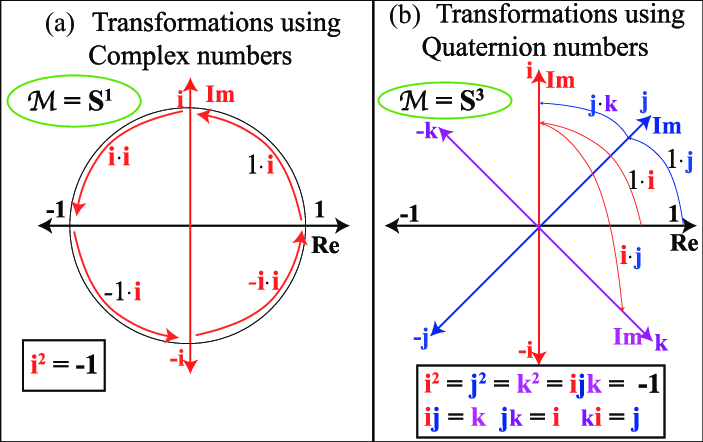Note: √-1 × √-1 = √ (-1 × -1) = √1 = one contradicts the actual fact that i2 = -1. Therefore, for an imaginary number, √a × √b is not equal √ab.

## Complex Numbers Identities

Let us see several identities.

• (z1 + z2)2 = (z1)2 + (z2)2 + 2 × z1 × z2
• (z1 – z2)2 = (z1)2 + (z2)2 – 2 × z1 × z2
• (z1)2 – (z2)2 = (z1 + z2) (z1 – z2)
• (z1 + z2)3 = (z1)3 + 3 × (z1)2 × z2 +3 × (z2)2 × z1 + (z2)3
• (z1 – z2)3 = (z1)3 – 3 × (z1)2 × z2 +3 × (z2)2 × z1 – (z2)3

Properties of Complex Numbers:
The properties of complex numbers given below:

• The addition of 2 conjugate complex varieties can lead to an actual or real number.
• The multiplication of 2 conjugate {complex numbers} also will lead to an actual or real number.
• If x and y are the real numbers and x + yi =0, then x =0 and y =0
• If p, q, r, and are the real numbers and p + qi = r + si, then p = r, and q=s
• The complex quantity obeys the commutative law of addition and multiplication.

z1+z2 = z2+z1

z1 x z2 = z2 x z1

• The complex quantity obeys the associative law of addition and multiplication.

(z1+z2) +z3 = z1 + (z2+z3)

(z1 x z2) x z3 = z1 x (z2 x z3)

• The complex quantity obeys the distributive law

z1 x (z2+z3) = z1 x z2 + z1 x z3

• If the addition of 2 complex numbers is real, and the merchandise of 2 complex numbers is additionally real, then these complex numbers are conjugate to every difference.
• For any complex numbers, say z1 and z2, then |z1+z2| ≤ |z1|+|z2|
• The results of the multiplication of 2 complex varieties and its conjugate worth ought to lead to a complex number ought to be a positive worth.

## Modulus and Conjugate

Let z = a + ib be a complex number.
The Modulus of z pictured by |z|. Mathematically, |z|=√ (a2+b2)The conjugation of z denotes by z¯. Mathematically, z¯= a – ib

### Argand Plane and Polar Representation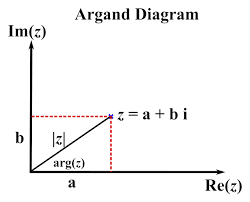We all apprehend that the pair of numbers (x, y) described on the XY-plane, where x is called coordinate, y is called the ordinate. Similarly, we will represent complex numbers conjointly on a plane called an Argand plane or complex plane. Similar to the coordinate-axis and ordinate-axis in two-dimensional geometry, there are two axes in the Argand plane.

• The horizontal axis is called the real or actual axis
• The vertical axis is called the imaginary or fanciful axis

The complex number x +iy that coincides with the ordered pair (x, y) is described geometrically as the distinctive point (x, y) within the XY-plane.
For example,
The complex number, 3 + 2 (i), corresponds to the ordered pair (3, 2) geometrically.
Similarly, -2+3i corresponds to the ordered pair (-2, 3).

• Complex numbers in the form 0+ai, where a is any real numbers will lie on the fanciful axis.
• Complex numbers in the form a+0i, where a is any real numbers will lie on the actual axis.

It's clear that the Modulus of complex number x +iy, √ (x2+y2) is that the distance between the origin (0, 0) and the distinctive point (x, y).

• The conjugate of z = x +iy is z = x -iy described as (x, -y) in the Argand plane. Point (x, -y) is the similarity of the distinctive point (x, y) across the real axis in the Argand plane.

Example: Find the distance between the complex number z = 4 – 3i and the origin in the Argand plane.
Distance between the origin and z= 3 – 4i is equal to the Modulus of z.
|z| = √ (42+(−3)2 ) = √ (16+9) = √ 25 = 5 units

### Polar illustration of complex numbers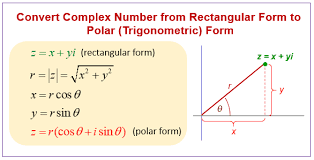Let A represent the non-zero complex number x + iy. OA is the directed line segment of length r and makes an angle θ with the positive direction of the coordinate-axis.
The ordered pair (r, θ) called the polar coordinates of point A, as point A is unambiguously determined by (r, θ). The origin is called the pole and the positive coordinate-axis is called the initial line.
Then,
x = r cosθ
y = r sinθ
We can write z = x + iy as z = r cosθ + ir sinθ = r(cosθ + i sinθ), which is named to be a polar variety of complex numbers.

• Here, r = |z| = √ (x2+y2) is the Modulus of z, θ known as the argument or amplitude of z denoted as arg z
• For any non-zero complex number z, there corresponds one value of θ, in the interval [0, 2π)
• In any other interval of length 2π, for instance, consider the -π < θ ≤ π, then the value of θ is called the principal argument of z.

## Solved Examples

 Example 1

Represent z = √3 + i in the polar form

Solution:

Given z =  √3 + i

Polar form  is  z =  rcosθ + r isinθ

equating the real and inmaginary part above

√3 + i = rcosθ + r isinθ

√3  = rcosθ   -- (a)

squaring both sides we have

3 = r2cos2θ --(1)

i  = r isinθ ==> 1 = rsinθ -- (b)

squaring both sides

1 = r2sin2θ---(2)

adding 1 and 2

we have

3 +1 = r2cos2θ + r2sin2θ

4 =  r [Since cos2θ +sin2θ = 1]

==> r = 2

substituting r = 2 in (a) and (b)  we have

√3  = 2 cosθ  ;  1 = 2sinθ

cosθ = √3 /2  ;  sinθ = 1/2

since sinθ and cosθ both are positive aargument will be I quadrant  and since cosθ = √3 /2  ;  sinθ = 1/2   ; θ = 30°

Argument = 30 × π/180 = π//6

now r = 2  and θ = π//6
Therefore, polar form of z is,
z = 2 (cos (π/6) + i sin (π/6))

 z = 2 (cos (π/6) + i sin (π/6))

## Summary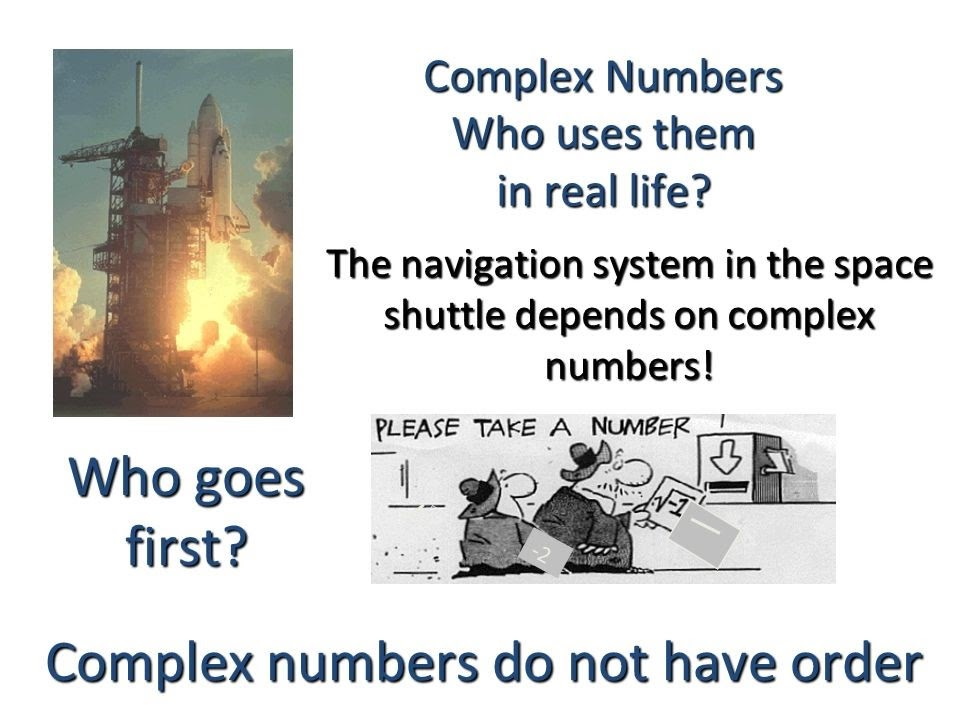You have got to recollect the following:
Every real number may be a complex number, but every complex number is not essential to the Real number.
The set of all complex numbers denoted by Z = CZ∈C.
The set of all imaginary numbers denoted as Z = C - b RZ∈C−R.

## 1. What is meant by complex numbers?

The complex number is the combination of a true or a real number and an imaginary number. An example of a complex number is 4+3i. Here 4 is a Real number 3i is a complex number.

## 2. Write down the increasing identity and inverse of the complex number?

The increasing identity of complex numbers outlined as (x +yi). (1+0i) = x +yi. Hence, the increasing identity is 1+0i. The increasing identity of complex numbers outlined as (x +yi) x (1/x +yi) = 1+0i. Hence, the increasing identity is 1/x +yi.

## 3. How to divide the complex numbers?

To divide the complex quantity, multiply the dividend and also the divisor by its conjugation. The conjugation of the Complex number is a dynamic sign between the two terms within the value of the divisor. Then apply the FOIL technique to change the expression.

## 4. Mention the arithmetic rules for complex numbers?

The arithmetic rules of complex numbers are:
Addition Rule: (a +bi) + (c +di) = (a +c) + (b +d)i
Subtraction Rule: (a +bi) – (c +di) = (a-c) + (b-d)i
Multiplication Rule: (a +bi) x (c +di) = (ac-bd) + (ad +bc)i

## 5. Write down the additive identity and inverse of complex numbers?

The additive identity of complex numbers is written as (x +yi) + (0+0i) = x +yi. Hence, the additive identity is 0 + 0i. The opposite (inverse) of complex numbers is written as (x +yi)+ (-x-yi) = (0+0i). Hence, the opposite is -x-yi.

Written by Priti Jain, Cuemath Teacher

Related Articles
GIVE YOUR CHILD THE CUEMATH EDGE
Access Personalised Math learning through interactive worksheets, gamified concepts and grade-wise courses
Learn More About Cuemath